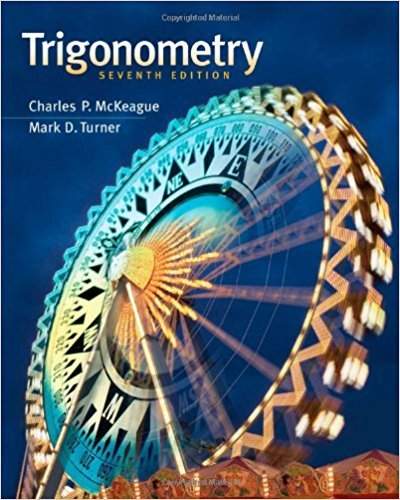×
×

# Solutions for Chapter 1-8: Complex Numbers and Polar Coordinates## Full solutions for Trigonometry | 7th Edition

ISBN: 9781111826857Solutions for Chapter 1-8: Complex Numbers and Polar Coordinates

Solutions for Chapter 1-8
4 5 0 342 Reviews
21
3
##### ISBN: 9781111826857

Trigonometry was written by and is associated to the ISBN: 9781111826857. This expansive textbook survival guide covers the following chapters and their solutions. Chapter 1-8: Complex Numbers and Polar Coordinates includes 1 full step-by-step solutions. This textbook survival guide was created for the textbook: Trigonometry, edition: 7. Since 1 problems in chapter 1-8: Complex Numbers and Polar Coordinates have been answered, more than 24677 students have viewed full step-by-step solutions from this chapter.

Key Math Terms and definitions covered in this textbook
• Associative Law (AB)C = A(BC).

Parentheses can be removed to leave ABC.

• Augmented matrix [A b].

Ax = b is solvable when b is in the column space of A; then [A b] has the same rank as A. Elimination on [A b] keeps equations correct.

• Characteristic equation det(A - AI) = O.

The n roots are the eigenvalues of A.

• Diagonalizable matrix A.

Must have n independent eigenvectors (in the columns of S; automatic with n different eigenvalues). Then S-I AS = A = eigenvalue matrix.

• Factorization

A = L U. If elimination takes A to U without row exchanges, then the lower triangular L with multipliers eij (and eii = 1) brings U back to A.

• Gauss-Jordan method.

Invert A by row operations on [A I] to reach [I A-I].

• Hessenberg matrix H.

Triangular matrix with one extra nonzero adjacent diagonal.

• lA-II = l/lAI and IATI = IAI.

The big formula for det(A) has a sum of n! terms, the cofactor formula uses determinants of size n - 1, volume of box = I det( A) I.

• Network.

A directed graph that has constants Cl, ... , Cm associated with the edges.

• Norm

IIA II. The ".e 2 norm" of A is the maximum ratio II Ax II/l1x II = O"max· Then II Ax II < IIAllllxll and IIABII < IIAIIIIBII and IIA + BII < IIAII + IIBII. Frobenius norm IIAII} = L La~. The.e 1 and.e oo norms are largest column and row sums of laij I.

• Normal matrix.

If N NT = NT N, then N has orthonormal (complex) eigenvectors.

• Nullspace matrix N.

The columns of N are the n - r special solutions to As = O.

• Orthonormal vectors q 1 , ... , q n·

Dot products are q T q j = 0 if i =1= j and q T q i = 1. The matrix Q with these orthonormal columns has Q T Q = I. If m = n then Q T = Q -1 and q 1 ' ... , q n is an orthonormal basis for Rn : every v = L (v T q j )q j •

• Particular solution x p.

Any solution to Ax = b; often x p has free variables = o.

• Pascal matrix

Ps = pascal(n) = the symmetric matrix with binomial entries (i1~;2). Ps = PL Pu all contain Pascal's triangle with det = 1 (see Pascal in the index).

• Polar decomposition A = Q H.

Orthogonal Q times positive (semi)definite H.

• Reflection matrix (Householder) Q = I -2uuT.

Unit vector u is reflected to Qu = -u. All x intheplanemirroruTx = o have Qx = x. Notice QT = Q-1 = Q.

• Right inverse A+.

If A has full row rank m, then A+ = AT(AAT)-l has AA+ = 1m.

• Similar matrices A and B.

Every B = M-I AM has the same eigenvalues as A.

• Singular matrix A.

A square matrix that has no inverse: det(A) = o.

×